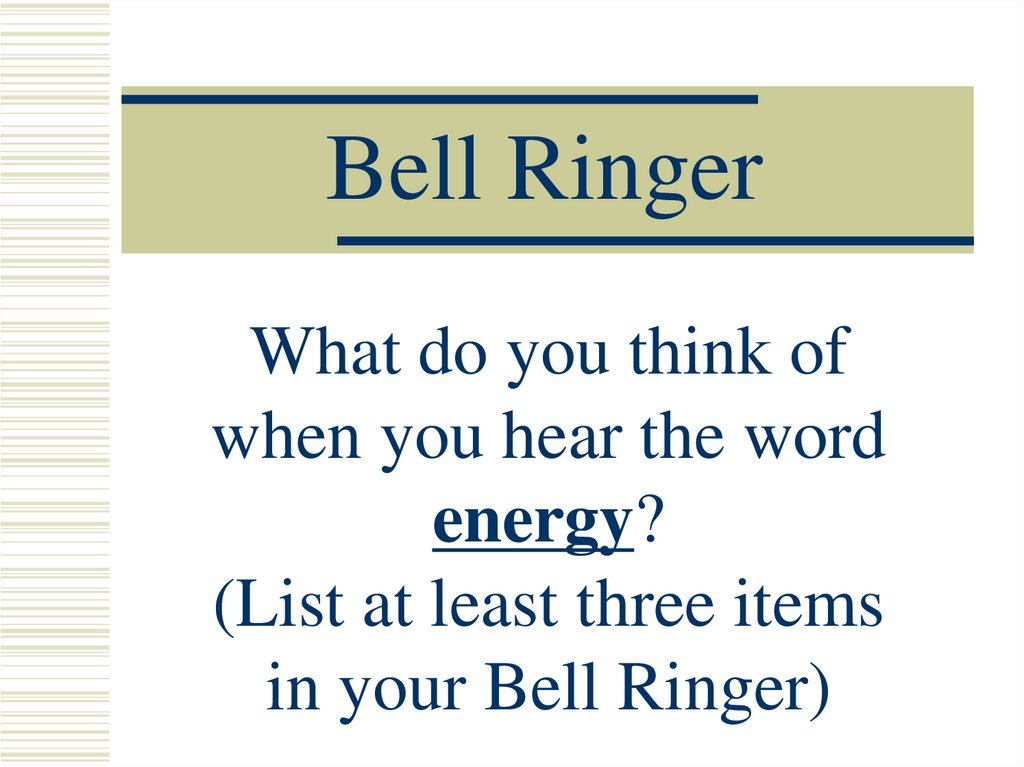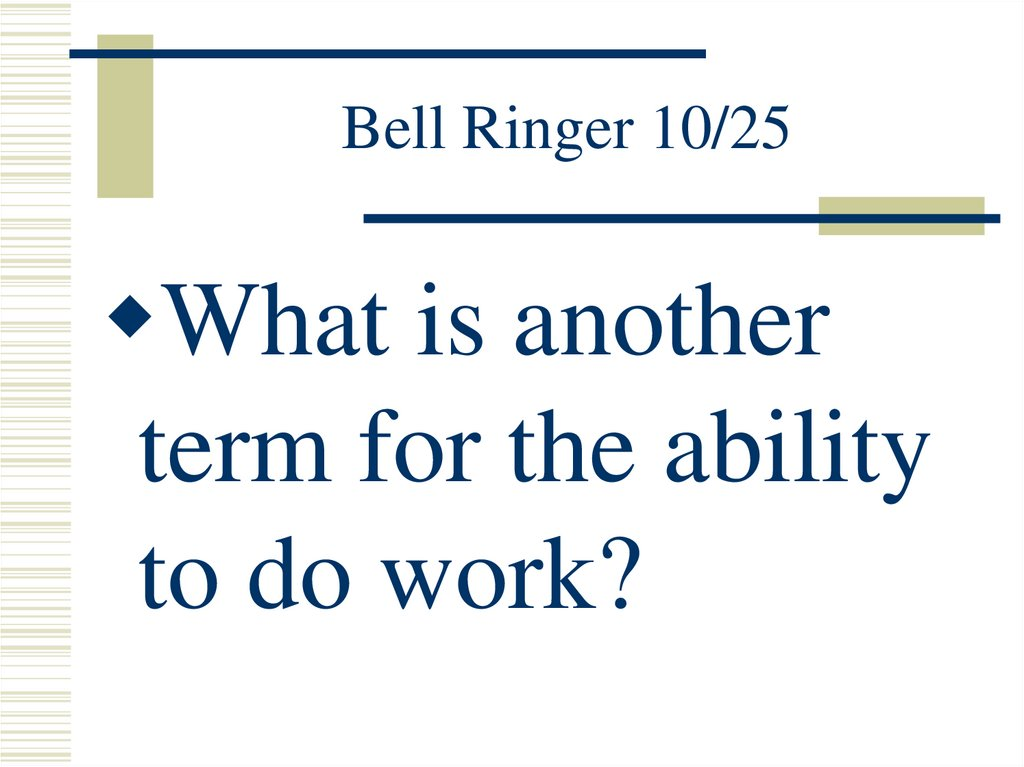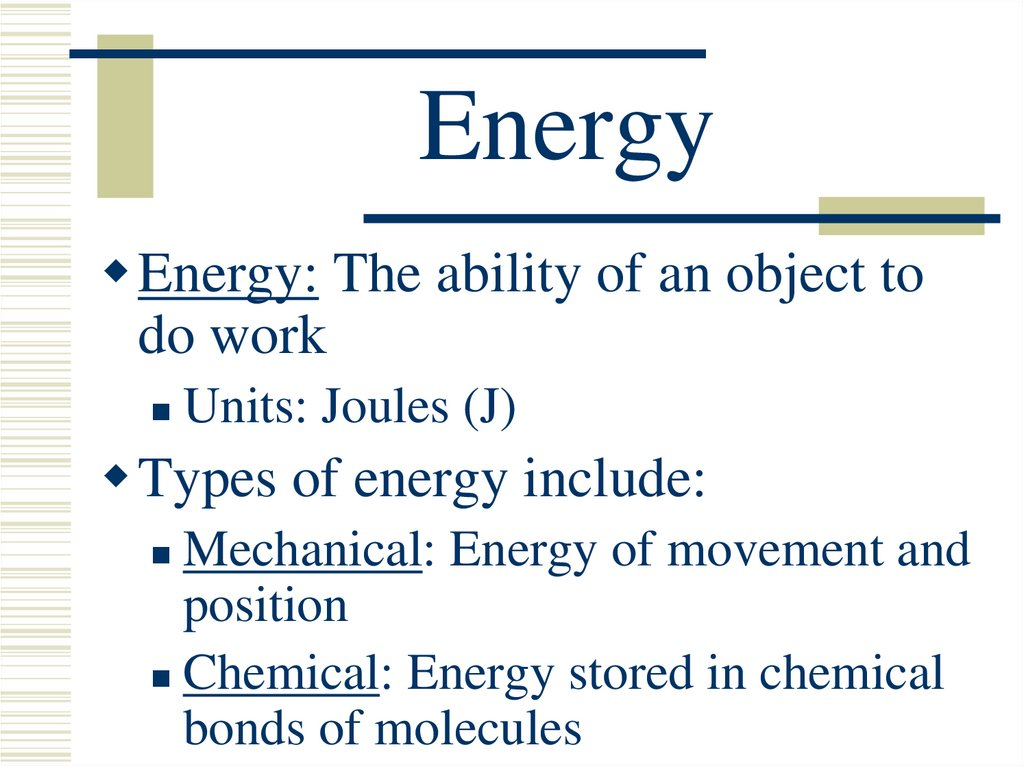# Bell Ringer. What do you think of when you hear the word energy?

## 1. Bell Ringer

What do you think of
when you hear the word
energy?
(List at least three items

## 2. Bell Ringer 10/25

What is another
term for the ability
to do work?

## 3. Energy

Energy: The ability of an object to
do work
Units: Joules (J)
Types of energy include:
Mechanical: Energy of movement and
position
Chemical: Energy stored in chemical
bonds of molecules

## 4. Energy

Thermal: “Heat energy” stored in
materials at a certain temperature
Nuclear: Energy produced from the
splitting of atoms
form of electromagnetic waves
Electric Energy: Energy traveling as
the flow of charged particles
(i.e. electrons)

## 5. Work

Work is done when a task produces
a change in energy
Factors affecting work done:
The application of a force
The movement of the object by that
force over a distance

## 6. Bell Ringer

How much work is
required to lift a 2kg
object 2m high?

## 7. Work

Therefore:
Work = Force x Distance
W = Fd
Units: Joule (J)
1 J = 1 N.m
Note that work requires a distance

## 8. Bell Ringer 3/31

What is another term for the
ability to do work?
You push a stationary wall with
a force of 1000N. How much
work was done to the wall?

## 10. Power

How much work is performed
over a period of time
Therefore:
Power = Work / Time
P = W/t
Units: Watts (W) where 1 W = 1 J/s

How many
horses are in
one
horsepower?

## 12. Power

Power can also be converted to
units of horsepower (hp)
Note: 1 hp 750 W
coffee maker
blender
lawn mower
Corvette
0.75 hp
1.5 hp
5-6 hp
400 hp

## 13. Bell Ringer

If Superman, at 90kg, jumps a
40m building in a single bound,
how much does Superman
perform?
If this occurs in 3s, what is his
power output?

## 14. Energy

The amount of work done by an
object does not depend on the path
taken
Work depends only on the object’s
starting and ending points
As work is done on an object, the
object itself gains the opportunity to
do work

## 15. Energy

For example:
A bowstring drawn back on a bow
Winding an alarm clock
Raising the arm on a pile driver
All of these objects now have
the ability to do work

## 16. Mechanical Energy

Mechanical Energy: Energy
of movement and position
There are two major types of
mechanical energy:
Potential
Energy: Energy of
position
Kinetic Energy: Energy of motion

## 17. Potential Energy

Gravitational Potential Energy:
The potential due to elevated
positions
P.E. = mass x gravity x height
P.E. = mgh
Recall: weight = mass x gravity
Therefore: P.E. = weight x height

## 19. Kinetic Energy

Objects in motion are capable of
doing work
.
.
2
KE = ½ mass velocity
KE =
2
½mv

## 20. Kinetic Energy

Note that the velocity of the object
is squared when determining KE
If the velocity of the object is

## 21. Energy Conservation

Energy is constantly
transforming, but never
“disappears”
Law of Conservation of Energy:
Energy cannot be created or
destroyed, only changed from
one form to another.

## 22. Energy Conservation

Potential and kinetic energy are
constantly transforming back and
forth
Most of the time during this
transformation, some energy is turned
to heat and transferred out of the system

## 25. Bell Ringer

Jill has a velocity of 5m/s. If she has a mass
of 60kg, what is her kinetic energy?
If Bob, at 70kg, is standing on top of a 13m
high hill. What is his potential energy?

## 26. Work-Energy Theorem

The change in gravitational
potential energy of an object is
equal to the amount of work
needed to change its height
Therefore:
Work = DPE
Fd = mgh

## 27. Work-Energy Theorem

The KE of a moving object is
equal to the work the object is
capable of doing while being
brought to rest
Therefore:
W = DKE or Fd =
2
½mv

## 28. Work-Energy Theorem

Putting these two ideas together gives
us the general Work-Energy
Theorem:
If no change in energy occurs,
then no work is done. Therefore,
whenever work is done, there is
a change in energy.

List and give an
example of the 6
types of simple
machines.

## 30. Simple Machines

Machine: A device used to multiply
forces or to change the directions of
forces
There are six types of simple
machines:
Pulley: Grooved wheels which assist in
raising, lowering, or moving an object

## 31. Simple Machines

Lever: A stiff bar which pivots on a
support to assist in lifting or moving an
object
Wedge: An object consisting of a
slanting side ending in a sharp edge
which separates or cuts materials apart
Wheel and Axle: A wheel with a rod
through its center which lifts or moves
objects

## 32. Simple Machines

Inclined Plane: A slanting surface
connecting a lower level to a higher
level
Screw: An inclined plane wrapped
around a rod which holds objects
together or lifts materials

## 33. Bell Ringer

What is an example
of a 100% efficient
machine?

of output force to input force
Input Force
i.e. A machine which outputs 80 N for every
10 N you put in has a mechanical advantage
of 8.
Note that the load will move only 1/8 of the
input distance

## 35. Efficiency

Efficiency: A machine’s ratio of
useful work output to total work input
Efficiency = Useful Work Output
Total Work Input
Efficiency is expressed as a percent
i.e.) An efficiency result of 0.25 means
25% efficiency

## 36. Efficiency

Ideal machines have 100% efficiency
This means that all of the energy put
into the machine exits as useful energy
All other machines will ALWAYS
have an efficiency of less than 100%
A machine cannot output more
work than is put into it

## 37. Pulleys

Single Pulley:
Changes the direction of a force
exerted by a rope or cable
System of pulleys:
Multiplies input forces, creating
large output forces

## 38. Pulleys

• Each supporting strand of rope holds an
equal fraction of the weight
• Tension in this cable is the same
throughout its entire length
• Input force = tension in each
supporting segment of the cable
• Mechanical advantage = number of
supporting strands

## 39. Pulleys

Input force = 30 N
30 N

## 40. Pulleys

Input force = 15 N
30 N

## 41. Bell Ringer

How many supporting
strands are there ?
What is the
here equal to?
What is the input
force required to lift
the 200kg object?

## 42. More Practice

What is the minimum effort that
must be applied to lift the load?
For every 2 meters the rope is
pulled through what height does
the load rise off the ground?
What is the mechanical

LEVERS

## 44. Levers

A simple machine made of a bar
which turns about a fixed point
Fulcrum: The pivot point of a lever
Change the direction of or
multiply input forces

## 45. Three Types of Levers

Type 1 Lever: Fulcrum lies between
the input force and the load
i.e.) A seesaw
Type 2 Lever: The load lies
between the fulcrum and the input
force
i.e.) A pry bar

## 46. Three Types of Levers

Type 3 Lever: The input force lies
between the fulcrum and the load

## 48. Levers

If friction is small enough to neglect:
Work Input = Work Output
or
(Fd)input = (Fd)output
Therefore: A small input force over a
large distance will output a large
force over a small distance

## 51. Wedge

Wedge: An object consisting of a
slanting side ending in a sharp edge
which separates or cuts materials
apart
i.e.
knife

## 52. Wheel and Axel

Wheel and Axle: A wheel with a rod
through its center which lifts or
moves objects
ie: Cart

## 53. Inclined Plane

Inclined Plane: A slanting surface
connecting a lower level to a higher
level
i.e.
Accessibility ramp

## 54. Screw

Screw: An inclined plane wrapped
around a rod which holds objects
together or lifts materials

## 55. Compound Machine

Compound machines use two or simple